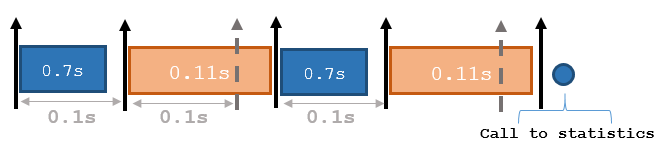# statistics

Statistics of past execution periods

## Syntax

``stats = statistics(rate)``

## Description

example

````stats = statistics(rate)` returns statistics of previous periods of code execution. `stats` is a struct with these fields: `Periods`, `NumPeriods`, `AveragePeriod`, `StandardDeviation`, and `NumOverruns`.```

Here is a sample execution graphic using the default setting, `'slip'`, for the `OverrunAction` property in the `Rate` object. See `OverrunAction` for more information on overrun code execution.The output of `statistics` is:

```stats = Periods: [0.7 0.11 0.7 0.11] NumPeriods: 4 AveragePeriod: 0.09 StandardDeviation: 0.0231 NumOverruns: 2```

## Input Arguments

expand all

`Rate` object, specified as an object handle. This object contains the information for the `DesiredRate` and other info about the execution. See `rateControl` (Navigation Toolbox) for more information.

## Output Arguments

expand all

Time execution statistics, returned as a structure. This structure contains the following fields:

• `Period` — All time periods (returned in seconds) used to calculate statistics as an indexed array. `stats.Period(end)` is the most recent period.

• `NumPeriods` — Number of elements in `Periods`

• `AveragePeriod` — Average time in seconds

• `StandardDeviation` — Standard deviation of all periods in seconds, centered around the mean stored in `AveragePeriod`

• `NumOverruns` — Number of periods with overrun

## Examples

expand all

Create a `rateControl` object for running at 20 Hz.

`r = rateControl(20);`

Start a loop and control operation using the `rateControl` object.

```for i = 1:30 % Your code goes here waitfor(r); end```

Get `Rate` object statistics after loop operation.

`stats = statistics(r)`
```stats = struct with fields: Periods: [0.0533 0.0485 0.0504 0.0494 0.0562 0.0424 0.0504 ... ] NumPeriods: 30 AveragePeriod: 0.0500 StandardDeviation: 0.0019 NumOverruns: 0 ```

## Version History

Introduced in R2016a